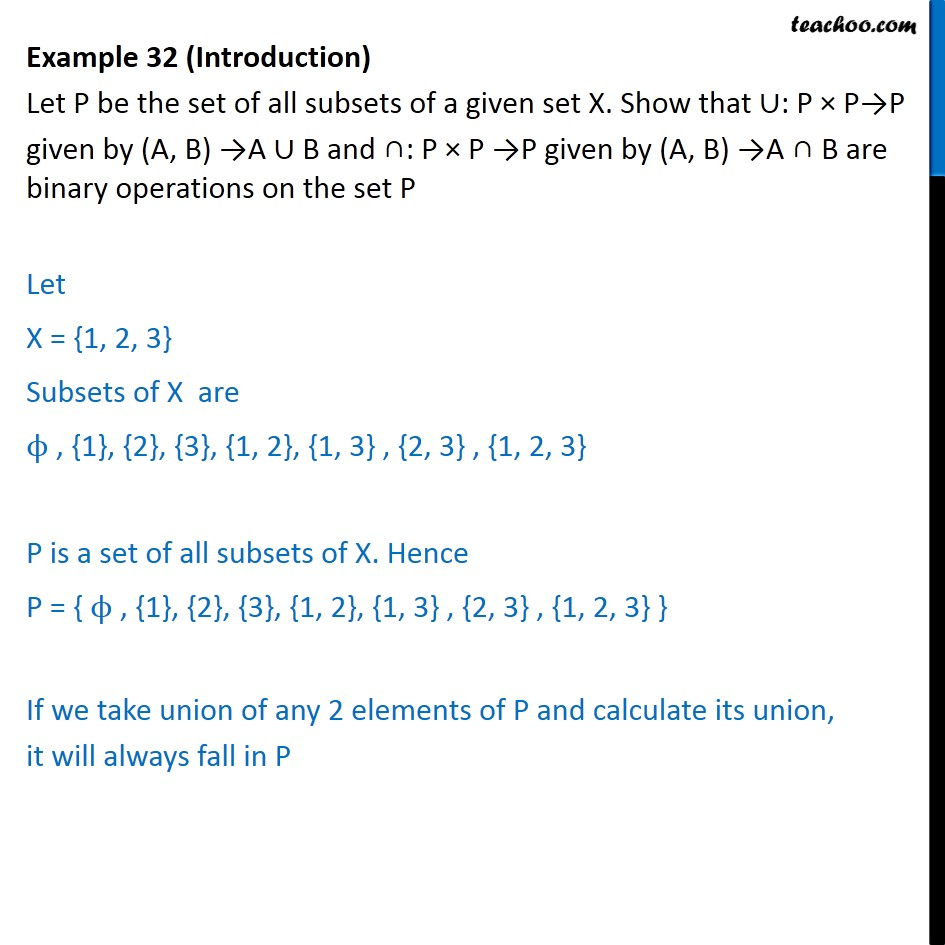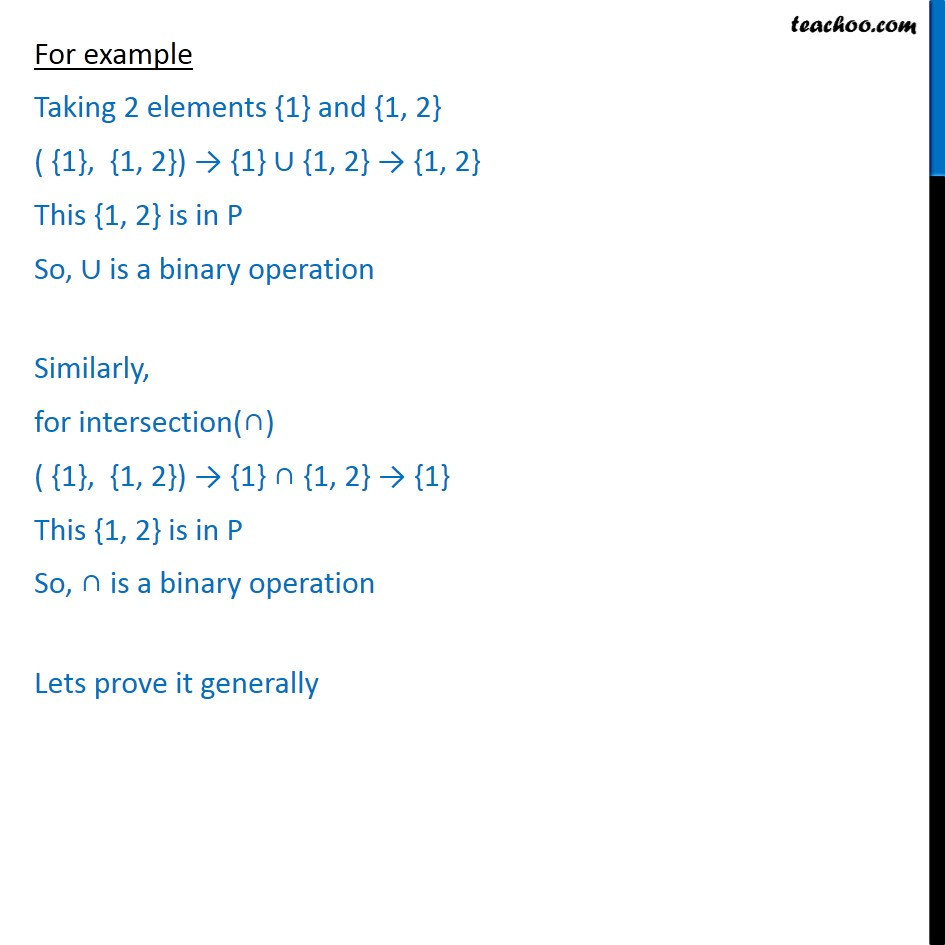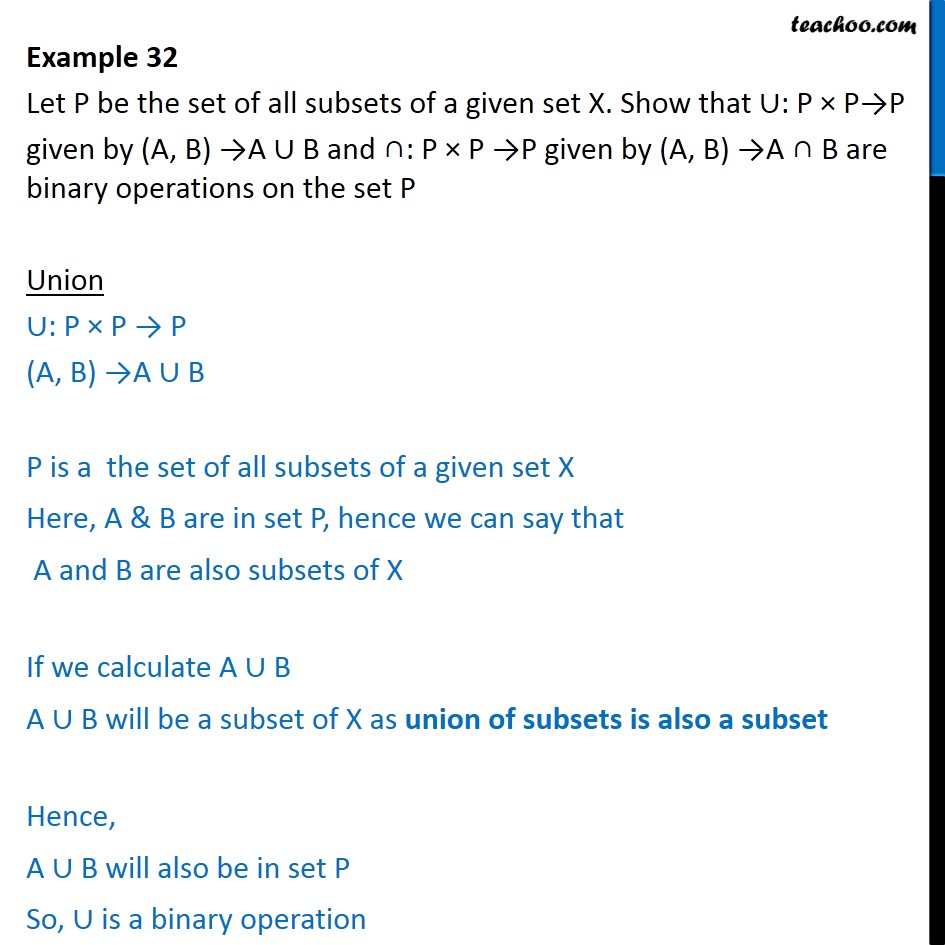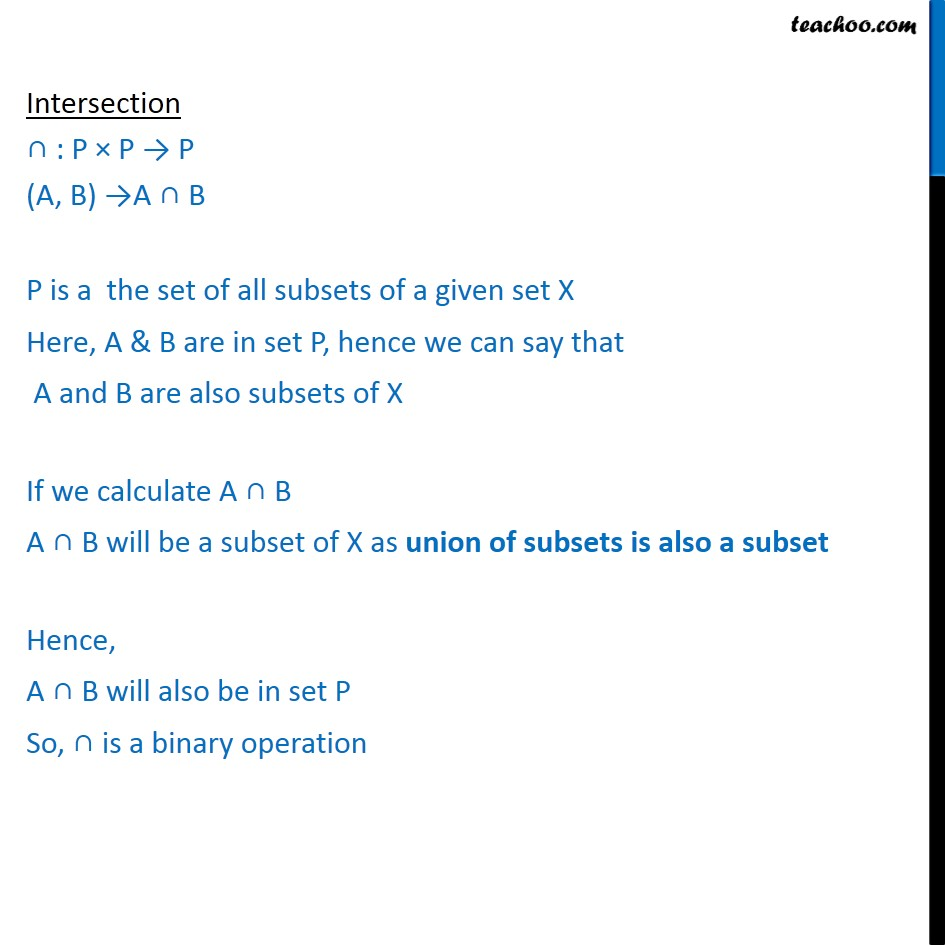Examples

Chapter 1 Class 12 Relation and Functions
Serial order wiseLearn in your speed, with individual attention - Teachoo Maths 1-on-1 Class

### Transcript

Question 15(Introduction) Let P be the set of all subsets of a given set X. Show that : P P P given by (A, B) A B and : P P P given by (A, B) A B are binary operations on the set P Let X = {1, 2, 3} Subsets of X are , {1}, {2}, {3}, {1, 2}, {1, 3} , {2, 3} , {1, 2, 3} P is a set of all subsets of X. Hence P = { , {1}, {2}, {3}, {1, 2}, {1, 3} , {2, 3} , {1, 2, 3} } If we take union of any 2 elements of P and calculate its union, it will always fall in P For example Taking 2 elements {1} and {1, 2} ( {1}, {1, 2}) {1} {1, 2} {1, 2} This {1, 2} is in P So, is a binary operation Similarly, for intersection( ) ( {1}, {1, 2}) {1} {1, 2} {1} This {1, 2} is in P So, is a binary operation Lets prove it generally Example 32 Let P be the set of all subsets of a given set X. Show that : P P P given by (A, B) A B and : P P P given by (A, B) A B are binary operations on the set P Union : P P P (A, B) A B P is a the set of all subsets of a given set X Here, A & B are in set P, hence we can say that A and B are also subsets of X If we calculate A B A B will be a subset of X as union of subsets is also a subset Hence, A B will also be in set P So, is a binary operation Intersection : P P P (A, B) A B P is a the set of all subsets of a given set X Here, A & B are in set P, hence we can say that A and B are also subsets of X If we calculate A B A B will be a subset of X as union of subsets is also a subset Hence, A B will also be in set P So, is a binary operation